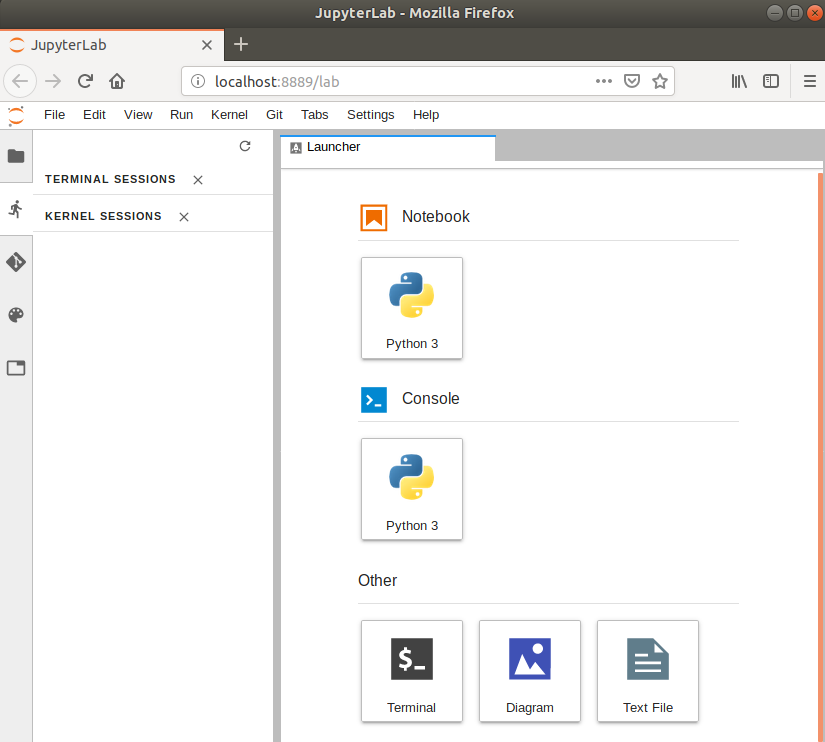This lesson is being piloted (Beta version)

# Introduction

## Overview

Teaching: 30 min
Exercises: 30 min
Questions
• What is jupyterlab?

• How to use jupyterlab?

• How to start and run simple python jupyter notebooks?

Objectives
• Get familiar with jupyterlab

• Run a simple jupyter notebook with python kernel

# What is Jupyterlab?

JupyterLab is the next-generation web-based user interface for Project Jupyter. It is made of modular building blocks:

• File explorer
• Text editor
• Diagram generator
• jupyter notebooks supporting more than 50 different Jupyter kernels
• Terminals
• Outputs

with an easy integration with Jupyterlab extension such as jupyterlab-latex for live-editing of LaTeX documents, jupyterlab-git for git integration or jupyterlab-drawio for creating diagrams.# Get familiar with Jupyterlab

Follow setup instructions and start `jupyterlab` from `anaconda-navigator`:## Create a new python 3 jupyter notebook

• Create a new python 3 jupyter notebook
``````from skimage import io
import matplotlib.pyplot as plt
%matplotlib inline

# https://directory.eoportal.org/web/eoportal/satellite-missions/c-missions/copernicus-sentinel-2
url="https://directory.eoportal.org/documents/163813/4091221/Sentinel2_Auto98.jpeg"
plt.imshow(image)
plt.title("Peruvian mountain scene, 14 July 2017, Sentinel-2\n (credit: ESA, processed by ESA, CC BY-SA 3.0 IGO)")
plt.show()
``````
• Rename your notebook to `example.ipynb`
``````# Using equation with LaTeX notation in a markdown cell

The well known Pythagorean theorem \$x^2 + y^2 = z^2\$ was
proved to be invalid for other exponents.
Meaning the next equation has no integer solutions:

\begin{equation}
x^n + y^n = z^n
\end{equation}
``````
``````import matplotlib
import matplotlib.pyplot as plt
import numpy as np

# Data for plotting
t = np.arange(0.0, 2.0, 0.01)
s = 1 + np.sin(2 * np.pi * t)

fig, ax = plt.subplots()
ax.plot(t, s)

ax.set(xlabel='time (s)', ylabel='voltage (mV)',
title='About as simple as it gets, folks')
ax.grid()

fig.savefig("test.png")
plt.show()
``````

Example above is taken from https://matplotlib.org/gallery/lines_bars_and_markers/simple_plot.html).

You can also add your own notebooks and try them out. Please note that some python packages may not be available in the provided environment.

You can get the list of available packages with the following command:

``````!conda list
``````

## Key Points

• jupyterlab

• jupyter notebook